# 90° Triangular Notch Weirs

This calculates the flow rate over a 90° triangular notch weir. The opening to this weir is a 90 degree triangular notch. The bottom of the notch is the lowest point with the sides going up at 45 degree angles. The water before the weir should be held in a relatively calm and smooth pool. There should be air underneath the water leaving the weir. The height is determined by measuring the ponded water elevation above the bottom of the weir. Learn more about the units used on this page.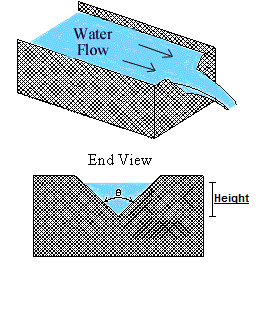Height:
Flow Rate

## The Equation

The equation to determine the flow rate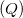of a 90° Triangular Notch Weir is: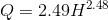Where: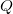= Flow Rate in cfs.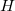= Height of the upstream water above the bottom of the weir in feet.

WSU Prosser - IAREC, 24106 N Bunn Rd, Prosser WA 99350-8694, 509-786-2226. Contact Us